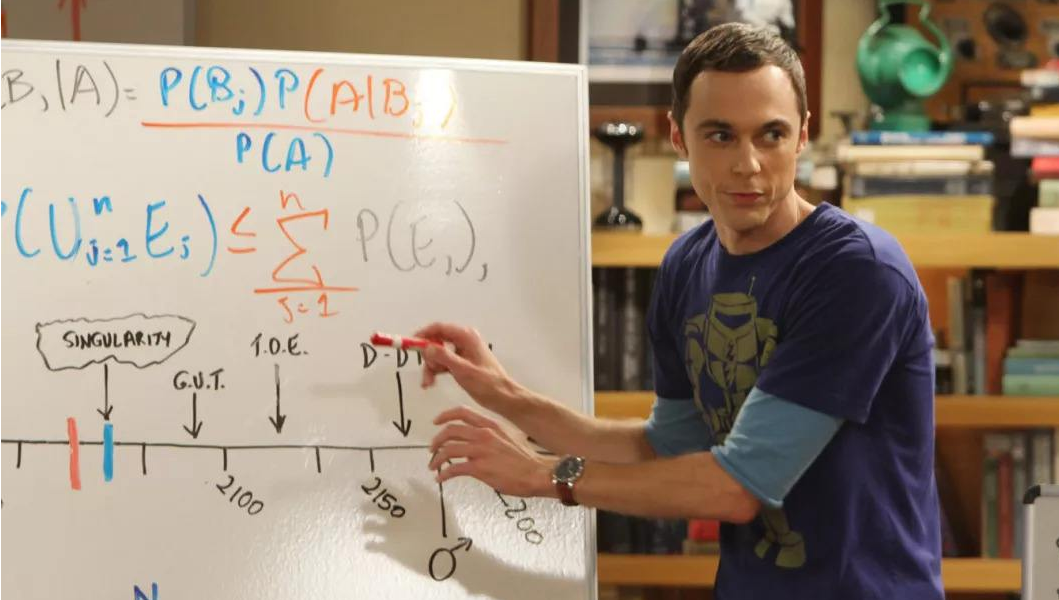## 先验与后验

P（拉肚子|喝凉水）——先验事件当中的条件概率

P（喝凉水|拉肚子）——后验概率

## 朴素贝叶斯概率

P（拉肚子）=P（喝凉水且拉肚子）+P（喝酸奶且拉肚子）
=P（喝凉水）*P（拉肚子|喝凉水）+P（喝酸奶）*P（拉肚子|喝酸奶）

P（喝凉水|拉肚子）=P（喝凉水且拉肚子）/P（拉肚子）
=P（喝凉水）*P（拉肚子|喝凉水）/P（拉肚子）
=P（喝凉水）*P（拉肚子|喝凉水）/P（喝凉水）*P（拉肚子|喝凉水）+P（喝酸奶）*P（拉肚子|喝酸奶）

## 贝叶斯的应用

P(B) = P(B|Ai) * P(Ai)
=P(B|A1) * P(A1) + P(B|A2) * P(A2) + P(B|A3) * P(A3)
=（500/1500*10%）+（400/1500*13%）+（600/1500*11%）
= 11.2%

P(A1|B)=P(A1) * P(B|A1) / P(B)
=（500/1500*10%）/ 11.2%
= 29.8%